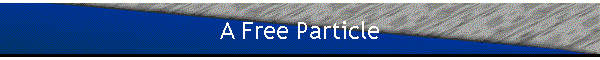Free particle solutions to the Schroedinger equation
A free particle is not subjected to any forces, its potential energy is constant.  Set U(r,t) = 0, since the origin of the potential energy may be chosen arbitrarily.
The Schroedinger equation then becomes  iħ∂Ψ(r,t)/∂t = -(ħ2/(2m))∇2Ψ(r,t).
Plane waves Ψ(r,t) = A exp(i(k∙r - ωt)) are possible solutions, as long as  ħω = ħ2k2/(2m).
We have
∂Ψ(r,t)/∂t = -iω Ψ(r,t),  ∇Ψ(r,t) = ik Ψ(r,t),  ∇2Ψ(r,t) = -k2Ψ(r,t),
ħωΨ(r,t) = (ħ2k2/(2m)) Ψ(r,t),  or E = p2/(2m)
using the de Broglie relations λ = h/p and f = E/h.

A plane wave represents a particle whose probability of presence is constant throughout space.
|Ψ(r,t)|2 = |A|2 = constant.
But a plane wave is not square-integrable, it is not a proper solution.
The Schroedinger equation is a linear equation, the principle of superposition applies.  A linear combination of plane wave solutions is also a solution.
Ψ(r,t) = ∑k ak exp(i(k∙r - ωkt)) will be a solution as long as for each k we have ħωk = ħ2k2/(2m).
Since k is a continuous variable, the most general solution is not a sum, but an integral.
Ψ(r,t) = (2π)-3/2∫g(k) exp(i(k∙r - ωkt)) d3k,   d3k = dkxdkydkz.
Such a wave function is called a three-dimensional wave packet and can represent any non-pathological square-integrable function.
(g(k) can be complex: g(k) = |g(k)|exp(iα(k)) ;  α(k) changes the phase of the plane wave.)
Proper wave functions of free particles are wave packets.